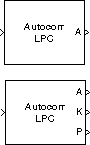# Autocorrelation LPC

Determine coefficients of Nth-order forward linear predictors

## Library

Estimation / Linear Prediction

`dsplp`

•## Description

The Autocorrelation LPC block determines the coefficients of an N-step forward linear predictor for the time-series in each length-M input channel, u, by minimizing the prediction error in the least squares sense. A linear predictor is an FIR filter that predicts the next value in a sequence from the present and past inputs. This technique has applications in filter design, speech coding, spectral analysis, and system identification.

The Autocorrelation LPC block can output the prediction error for each channel as polynomial coefficients, reflection coefficients, or both. It can also output the prediction error power for each channel. The input u can be an unoriented vector, column vector, or a matrix. Row vectors are not valid inputs. The block treats all M-by-N matrix inputs as N channels of length M.

When you select Inherit prediction order from input dimensions, the prediction order, N, is inherited from the input dimensions. Otherwise, you can use the Prediction order parameter to specify the value of N. Note that N must be a scalar with a value less than the length of the input channels or the block produces an error.

When Output(s) is set to `A`, port A is enabled. For each channel, port A outputs an (N+1)-by-1 column vector, a = [1 a2 a3 ... aN+1]T, containing the coefficients of an Nth-order moving average (MA) linear process that predicts the next value, ûM+1, in the input time-series.

`${\stackrel{^}{u}}_{M+1}=-\left({a}_{2}{u}_{M}\right)-\left({a}_{3}{u}_{M-1}\right)-...-\left({a}_{N+1}{u}_{M-N+1}\right)$`

When Output(s) is set to `K`, port K is enabled. For each channel, port K outputs a length-N column vector whose elements are the prediction error reflection coefficients. When Output(s) is set to `A and K`, both port A and K are enabled, and each port outputs its respective set of prediction coefficients for each channel.

When you select Output prediction error power (P), port P is enabled. The prediction error power is output at port P as a vector whose length is the number of input channels.

## Algorithm

The Autocorrelation LPC block computes the least squares solution to

`$\underset{i\in {\Re }^{n}}{\mathrm{min}}‖U\stackrel{˜}{a}-b‖$`

where $‖\cdot ‖$ indicates the 2-norm and

`$U=\left[\begin{array}{cccc}{u}_{1}& 0& \cdots & 0\\ {u}_{2}& {u}_{1}& \ddots & ⋮\\ ⋮& {u}_{2}& \ddots & 0\\ ⋮& ⋮& \ddots & {u}_{1}\\ ⋮& ⋮& ⋮& {u}_{2}\\ ⋮& ⋮& ⋮& ⋮\\ {u}_{M}& ⋮& ⋮& ⋮\\ 0& \ddots & ⋮& ⋮\\ ⋮& \ddots & \ddots & ⋮\\ 0& \cdots & 0& {u}_{M}\end{array}\right],\text{\hspace{0.17em}}\text{\hspace{0.17em}}\text{​}\text{​}\stackrel{˜}{a}=\left[\begin{array}{c}{a}_{2}\\ ⋮\\ {a}_{n}+1\end{array}\right],\text{\hspace{0.17em}}\text{\hspace{0.17em}}b=\left[\begin{array}{c}{u}_{2}\\ {u}_{3}\\ ⋮\\ {u}_{M}\\ 0\\ ⋮\\ 0\end{array}\right]$`

Solving the least squares problem via the normal equations

`${U}^{\ast }U\stackrel{˜}{a}={U}^{\ast }b$`

leads to the system of equations

`$\left[\begin{array}{cccc}{r}_{1}& {r}_{2}^{\ast }& \cdots & {r}_{n}^{\ast }\\ {r}_{2}& {r}_{1}& \ddots & ⋮\\ ⋮& \ddots & \ddots & {r}_{2}^{\ast }\\ {r}_{n}& \cdots & {r}_{2}& {r}_{1}\end{array}\right]\text{\hspace{0.17em}}\left[\begin{array}{c}{a}_{2}\\ {a}_{3}\\ ⋮\\ {a}_{n+1}\end{array}\right]\text{\hspace{0.17em}}=\left[\begin{array}{c}-{r}_{2}\\ -{r}_{3}\\ ⋮\\ -{r}_{n+1}\end{array}\right]$`

where r = [r1 r2 r3 ... rn+1]T is an autocorrelation estimate for u computed using the Autocorrelation block, and * indicates the complex conjugate transpose. The normal equations are solved in O(n2) operations by the Levinson-Durbin block.

Note that the solution to the LPC problem is very closely related to the Yule-Walker AR method of spectral estimation. In that context, the normal equations above are referred to as the Yule-Walker AR equations.

## Parameters

Output(s)

The type of prediction coefficients output by the block. The block can output polynomial coefficients (`A`), reflection coefficients (`K`), or both (```A and K```).

Output prediction error power (P)

When selected, enables port `P`, which outputs the output prediction error power.

Inherit prediction order from input dimensions

When selected, the block inherits the prediction order from the input dimensions.

Prediction order (N)

Specify the prediction order, N, which must be a scalar. This parameter is disabled when you select the Inherit prediction order from input dimensions parameter.

## References

Haykin, S. Adaptive Filter Theory. 3rd ed. Englewood Cliffs, NJ: Prentice Hall, 1996.

Ljung, L. System Identification: Theory for the User. Englewood Cliffs, NJ: Prentice Hall, 1987. Pgs. 278-280.

Proakis, J. and D. Manolakis. Digital Signal Processing. 3rd ed. Englewood Cliffs, NJ: Prentice-Hall, 1996.

## Supported Data Types

• Double-precision floating point

• Single-precision floating point

 Autocorrelation DSP System Toolbox Levinson-Durbin DSP System Toolbox Yule-Walker Method DSP System Toolbox `lpc` Signal Processing Toolbox

## Extended Capabilities

Introduced before R2006a

## Support평가판 신청